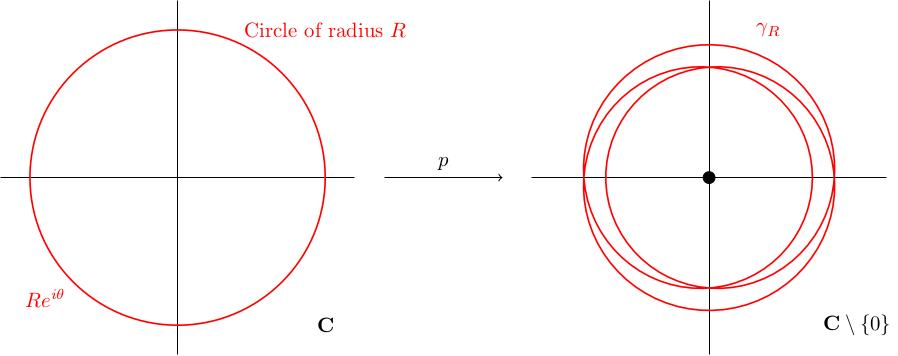# 1.01 Winding numbers and the fundamental theorem of algebra

Below the video you will find accompanying notes and some pre-class questions.

# Notes

(0.00) I want to start this module by giving a rough sketch of how to prove the fundamental theorem of algebra using the idea of winding numbers. You may have seen something similar in a first course on complex analysis, where the winding number was defined using a contour integral. The aim of the current proof is to remove the need for complex analysis in this definition: the winding number is something purely topological.

## The fundamental theorem of algebra

A nonconstant complex polynomial has a complex root.

(1.45) Let $$p\colon\mathbf{C}\to\mathbf{C}$$, $$p(z)=z^n+a_{n-1}z^{n-1}+\cdots+a_0$$, be a complex polynomial. Assume that $$p$$ has no complex root, in other words that there is no point $$x\in\mathbf{C}$$ for which $$p(x)=0$$. We will show that $$n=0$$, in other words that $$p$$ has only a constant term.

(2.24) Consider the circle of radius $$R$$ in the complex plane. The points in this circle are precisely those of the form $$Re^{i\theta}$$. Let $$\gamma_R$$ be the image of this circle under the map $$p\colon\mathbf{C}\to\mathbf{C}$$. We can think of $$\gamma_R$$ as a loop in $$\mathbf{C}$$: $\gamma_R(\theta)=p(Re^{i\theta}).$ Crucially, because $$p(z)\neq 0$$ for all $$z\in\mathbf{C}$$, the loop $$\gamma_R$$ is a loop in $$\mathbf{C}\setminus\{0\}$$.Figure 1. In this figure, we see the circle of radius $$R$$ in the domain of $$p$$ and its image $$\gamma_R$$ in the image of $$p$$ ($$\mathbf{C}\setminus\{0\}$$). In this example, $$p(z)=z^3-z/2$$ and $$R=2$$ and we see that the winding number of $$\gamma_R$$ is 3.

(3.54) When $$R=0$$, $$\gamma_0(\theta)=p(0)$$ is independent of $$\theta$$. In other words, $$\gamma_0$$ is the constant loop at the point $$p(0)\in\mathbf{C}\setminus\{0\}$$.

(4.42) When $$R$$ is very large, the term $$z^n$$ dominates in $$p$$, so $$\gamma_R(\theta)\approx \delta(\theta)$$, where $$\delta(\theta)=R^ne^{in\theta}$$.

Claim: (6.54) There is a homotopy invariant notion of winding number around the origin for paths in $$\mathbf{C}\setminus\{0\}$$ which gives zero for the constant loop and $$n$$ for the loop $$\delta(\theta)=R^ne^{in\theta}$$.

Homotopy invariant means, roughly, invariant under continuous deformations; in our situation, that means that the winding number of $$\gamma_R$$ around the origin should be independent of $$R$$. Since $$\gamma_0$$ has winding number zero and $$\gamma_R$$ has winding number $$n$$ for large $$R$$, this implies $$n=0$$.

## Outlook

(9.24) The rest of this module will be about defining this notion of winding number, the notion of homotopy and homotopy invariance, and generalising it to other spaces. In a more general setting, the spaces we're interested in (in this example $$\mathbf{C}\setminus\{0\}$$) will have an associated group (in this example the integers $$\mathbf{Z}$$) called the fundamental group and loops will have winding numbers'' which are elements of this group.

This will have many applications, including:

• the Brouwer fixed point theorem (any continuous map from the 2-dimensional disc to itself has a fixed point).
• the fact that a trefoil knot cannot be unknotted.
• the fact that the three Borromean rings cannot be unlinked from one another (despite the fact that they can be unlinked in pairs ignoring the third).

# Pre-class questions

1. Go through the rough sketch proof and highlight all the steps which seem to you not to be fully justified. We will discuss this in class, and I will call upon you for suggestions. Later in the module, we will revisit this proof and fill in all the gaps (hopefully to your satisfaction).